## Section II - The Integral Equations For Free Space

The NEC program uses both an electric-field integral equation (EFIE) and a magnetic-field integral equation (MFIE) to model the electromagnetic response of general structures. Each equation has advantages for particular structure types. The EFIE is well suited for thin-wire structures of small or vanishing conductor volume while the MFIE, which fails for the thin-wire case, is more attractive for voluminous structures, especially those having large smooth surfaces. The EFIE can also be used to model surfaces and is preferred for thin structures where there is little separation between a front and back surface. Although the EFIE is specialized to thin wires in this program, it has been used to represent surfaces by wire grids with reasonable success for far-field quantities but with variable accuracy for surface fields. For a structure containing both wires and surfaces the EFIE and HFIE are coupled. This combination of the EFIE and MFIE was proposed and used by Albertsen, Hansen, and Jensen at the Technical University of Denmark (ref. 9) although the details of their numerical solution differ from those in NEC. A rigorous derivation of the EFIE and MFIE used in NEC is given by Poggio dnd Hiller (ref. 10). The equations and their derivation are outlined in the following sections.

1. THE ELECTRIC FIELD INTEGRAL EQUATION (EFTE) The form of the EFIE used in NEC follows from an integral representation for the electric field of a volume current distribution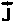, Equation(1)where Equation(1a)and the time convention is exp(jwt).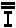is the identity dyad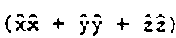. When the current distribution is limited to the surface of a perfectly conducting body, equation (1) becomes Equation (2)with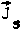the surface current density. The observation point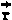is restricted to be off the surface S so that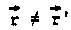.

Ifapproaches S as a limit, equation (2) becomes Equation (3)Where the principal value integral,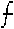, is indicated since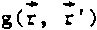is now unbounded.

An integral equation for the current induced on S by an incident field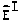can be obtained from equation (3) and the boundary condition for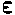S, Equation (4)where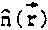is the unit normal vector of the surface atand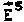is the field due to the induced current. Substituting equation (3) foryields the integral equation,Equation (5)

The vector integral in equation (5) can be reduced to a scalar integral equation when the conducting surface S is that of a cylindrical thin wire, thereby making the solution much easier. The assumptions applied for a thin wire, known as the thin-wire approximation, are as follows:

a. Transverse currents can be neglected relative to axial currents on the wire.

b. The circumferential variation in the axial current can be neglected.

c. The current can be represented by a filament on the wire axis.

d. The boundary condition on the electric field need be enforcrd in the axial direction only.

These widely used approximations are valid as long as the wire radius is much less than the wavelength and much less than the wire length. An alternate kernel for the EFIE, based on an extended thin-wire approximation in which condition c is relaxed, is also included in NEC for wires having too large a radius for the thin-wire approximation (ref. 11).

From assumptions a, b and c, the surface current() on a wire of radius a can be replaced by a filamentary current I where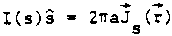s=distance parameter along the wire axis at, and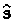=unit vector tangent to the wire axis at.

Equation (5) then becomes Equation6 where the integration is over the length of the wire. Enforcing the boundary condition in the axial direction reduces Eq. (6) to the scalar equation, Equation(7) Since' is now the point at s' on the wire axis whileis a point at s on the wire surface |-'|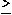a and the integrand is bounded.

2. THE MAGNETIC FIELD INIEGRAL EQUATION (MFIE) The MFIE is derived from the integral representation for the magnetic field of a surface current distribution. Equation (8) where the differentiation is with respect to the integration variable. If the currentis induced by an external incident field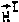, then the total magnetic field inside the perfectly conducting surface must be zero. Hence, forjust inside the surface S, Equation(9) whereis the incident field with the structure removed, and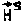is the scattered field given by equation (8). The integral equation formay be obtained by lettingapproach the surface point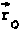from inside the surface along the normal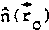· The surface component of equation (9) with equation (8) substituted foris then Equation (9a) whereis the outward directed normal vector at. The Limit can be evaluated by using a result of potential theory (ref. 12) to yield the integral equation Equation (10) For solution in NEC, this vector integral equation is resolved into two scalar equations along the orthogonal surface vectors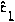and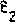where Equation (10a) By using the identity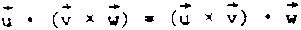and noting that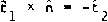and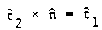, the scalar equation can be written, Equation (11) Equation (12) These two components suffice since there is no normal component of equation (10).

3. THE EFIE-MFIE HYBRID EQUATION Program NEC uses the EFIE for thin wires and the MFIE for surfaces. For a structure consisting of both wires and surfaces,in equation (7) is restricted to the wires, with the integral for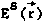, extending over the complete structure. The thin-wire form of the integral in equation (7) is used over wires while the more general form of equation (5) must be used on surfaces. Likewise,is restricted to surfaces in equations (11) and (12), with the integrals for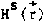extending over the complete structue. On wires the integral is simplified by the thin-wire approximation. The resulting coupled integral equations are, foron wire surfaces, Equation (13) and foron survaces excludeing wires Equation (13a) and Equation (13b) The symble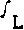represents integration over wires while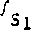represents intergration over survaces exluding wires. The numerical method used to solve equations (13), (14) and (15) is described in section III